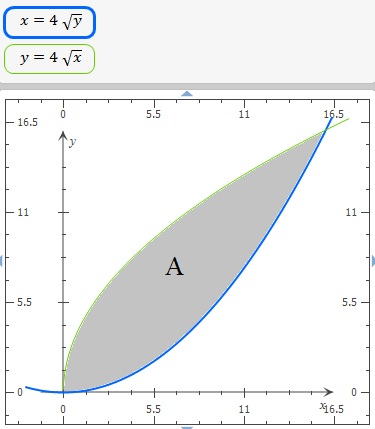# Find the bounderies given by the curve x = 4 sqrt(y) and y = 4 sqrt(x). Show your solution.

## Question:

Find the bounderies given by the curve {eq}x = 4 \sqrt y {/eq} and {eq}y = 4 \sqrt x {/eq}. Show your solution.

## Area Between Functions With Integration

In 2D calculus, the definite integral has many applications, for example, we can find the area between functions, we need the integration limits and solve the integral. Remember, the bigger area minus the smaller area.

The area is:The functions are:

{eq}\displaystyle \ f(x) = 4\sqrt{x} \\ {/eq}

and

{eq}\displaystyle x= 4\sqrt{y} \; \Rightarrow \; \frac{x^2}{16}=y \; \Rightarrow \; \ g(x)= \frac{x^2}{16} \\ {/eq}

Integration limits at interceptions {eq}x {/eq} values.

Matching functions:

{eq}\displaystyle \frac{x^2}{16}= 4\sqrt{x} \\ \displaystyle \frac{x^2}{16}- 4\sqrt{x}=0 \; \Leftrightarrow \; x=0 \; \text{or} \;x=16 \\ {/eq}

Terefore, the area is:

{eq}\displaystyle A \; = \; \displaystyle \int_{0}^{16} \ f(x) - \ g(x) \;dx \\ \displaystyle A \; = \; \displaystyle \int_{0}^{16} \ 4 \sqrt{x} - \frac{x^2}{16} \;dx \\ \displaystyle A \; = \; 8/3\,{x}^{3/2}-1/48\,{x}^{3} \bigg|_{0}^{16 } \\ \displaystyle A \; = \; 8/3\,{(16)}^{3/2}-1/48\,{(16)}^{3} - \left( 8/3\,{(0)}^{3/2}-1/48\,{(0)}^{3} \right) \\ \displaystyle A \; = \; \frac{256}{3} \; \text{or} \; A \; = \; 85.3333333333333 \; \; \text{ squared units } \; \\ {/eq}